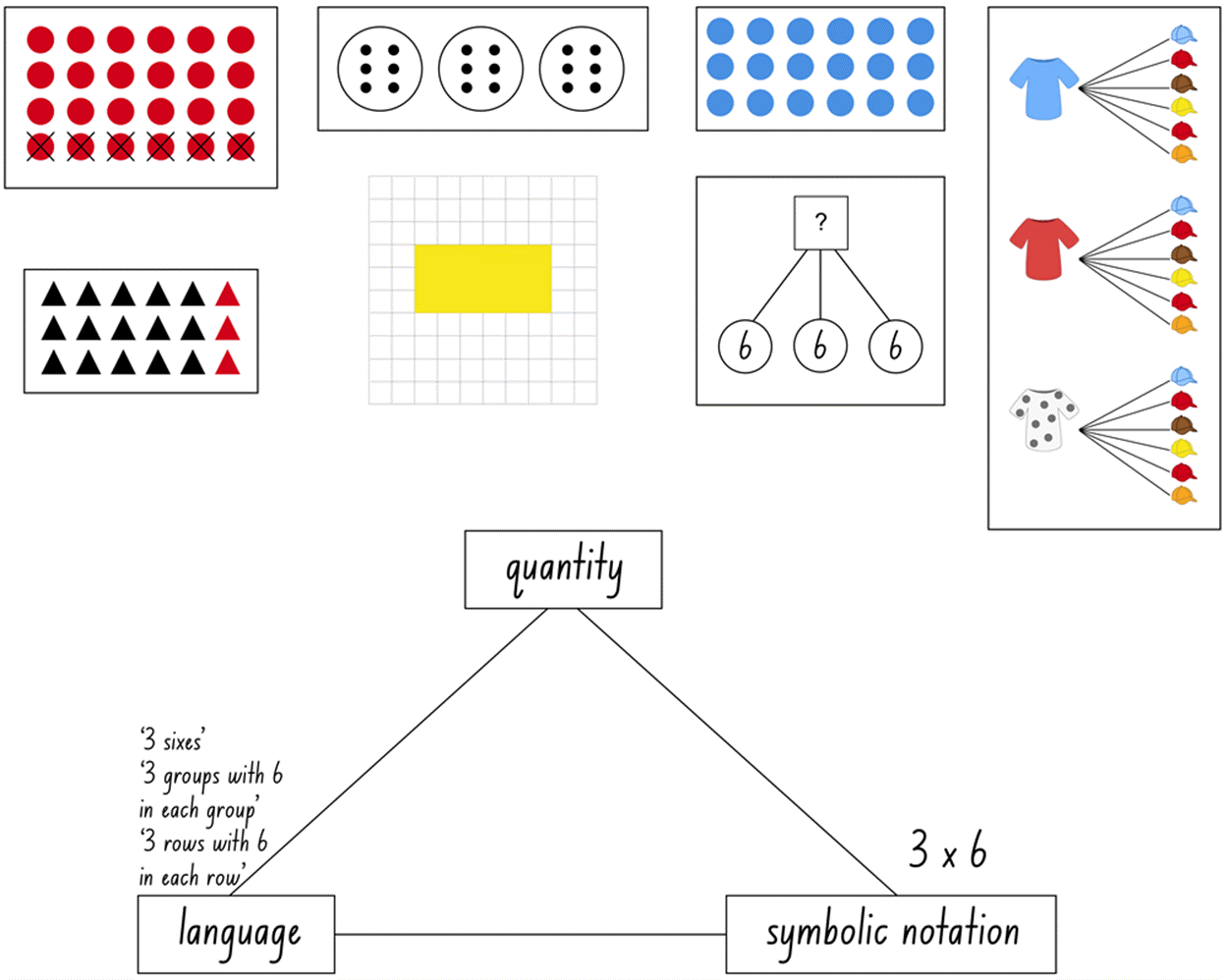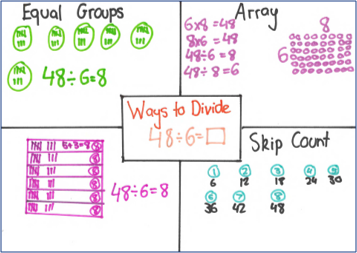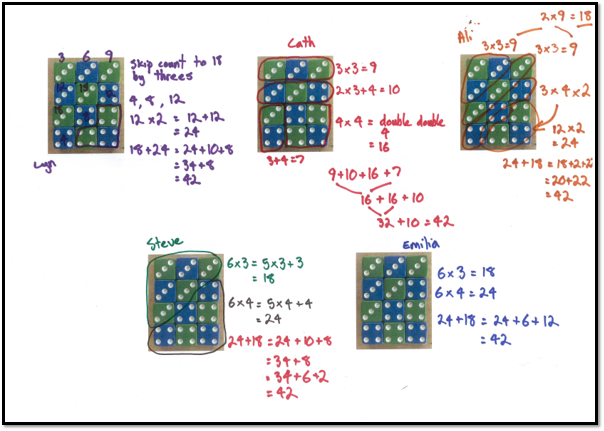# Multiplicative strategies

## What are multiplicative strategies?

Multiplicative strategies encompass a student’s ability to manipulate numbers in multiplicative situations. Students’ understanding of numbers as composite units and the ability to recognise and work with the relationship between quantities is a critical part of thinking multiplicatively. Students need to be able to understand the relationship between division and multiplication and develop the ability to flexibly use these as inverse operations when solving problems.

Professor Dianne Siemon describes multiplicative thinking as:

• a capacity to work flexibly and efficiently with an extended range of numbers (that is., larger whole numbers, decimals, common fractions, ratio and per cent),
• an ability to recognise and solve a range of problems involving multiplication or division including direct and indirect proportion, and
• the means to communicate this effectively in a variety of ways (for example, words, diagrams, symbolic expressions, and written algorithms).

Multiplicative strategies is a sub-element within the Number sense and algebra element of the National Numeracy Learning Progression.

Within the sub-elements of the numeracy progression, subheadings have been included to group indicators into particular categories of skills that develop over a number of levels. The seven subheadings within the Multiplicative strategies sub-element are:

• Forming equal groups (MuS1)
• Perceptual multiples (MuS2)
• Figurative (imagined units) (MuS3)
• Repeated abstract composite units (MuS4)
• Coordinating composite units (MuS5)
• Flexible strategies for multiplication/ Flexible strategies for division (MuS6)
• Flexible number properties (MuS7)

## Flexible strategies for multiplication

Number sense forms the foundation of all mathematical work. Having a ‘feel’ for numbers and understanding how to work flexibly and creatively with them is not just the domain of single units. In fact, being able to think flexibly about composite units is critical to all mental strategies for multiplication and division, laying the foundations for later mathematical concepts. Building strong number sense requires an understanding of numerical relationships, the use of strong mental imagery and the active making of connections between representations, situations, concepts, experiences and language.

(Adapted from Siemon, D., Beswick, K., Brady, K., Clark, J., Faragher, R., & Warren, E. (2015). Teaching mathematics. South Melbourne, Vic.: Oxford University Press.)Image: Various models of multiplication for 3 sixes and the connection between quantity, language and symbolic notation for 3 sixes.

## What are you noticing?

Here are classroom illustrations demonstrating flexible use of multiplicative strategies.Image: Student work sample showing flexible multiplicative strategies using a think boardImage: Work samples from five students showing the use of different strategies to find the total number of dots.

## Moving from concrete to abstract representations

This video explores the importance of navigating carefully from concrete to abstract representations of multiplicative situations to ensure students gain conceptual understanding (Stage 2).

Moving from concrete to abstract representations

## The role of talk

Kazemi and Hintz outline four key principles for classroom discussions about strategies. These principles are:

1. Discussions should achieve a mathematical goal
2. Students need to know what and how to share
3. Teachers need to orient students to one another and the mathematical ideas to achieve the mathematical goal
4. Teachers must communicate that all students are sense makers and that their ideas are valued.

Reference: Kazemi, E., & Hintz, A. (2014). Intentional Talk – How to structure and lead productive mathematical discussions. USA: Stenhouse Publishers.

They also highlight several targeted discussion routines that create productive discourse in the classroom.

The video below provides an explanation and example of the targeted discussion routine.

Troubleshoot and revise

## Professional learning

Category:

• Teaching and learning